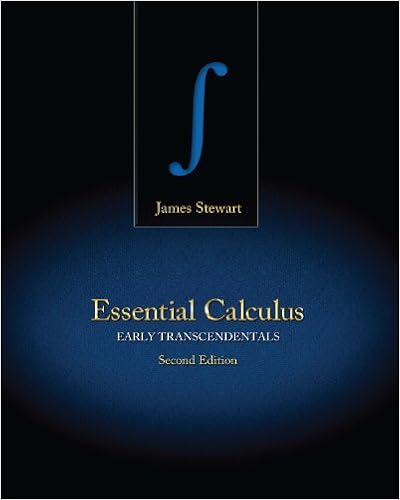# 4 gives z e 1 ln xdx x ln x j e 1 ze 1dx x ln xj e1 x

• Test Prep
• randy657
• 9

This preview shows page 3 - 6 out of 9 pages.

##### We have textbook solutions for you!
The document you are viewing contains questions related to this textbook.The document you are viewing contains questions related to this textbook.
Chapter 6 / Exercise 28
Essential Calculus: Early Transcendentals
StewartExpert Verified
Then, applying formula 4.4 givesZe1lnxdx=xlnxje1°Ze1dx=xlnxje1°xje1= (elne°ln 1)°(e°1)= 1Remark 298You will note that in the problem we just did, we found thatZlnxdx=xlnx°x+CExample 299FindRtan°1xdxIf we selectu= tan°1xdu=11 +x2dxv=xdv=dxThen, applying formula 4.4 givesZtan°1xdx=xtan°1x°Zx1 +x2dx(4.5)We will doRx1 +x2dxseparately, using the substitutionu= 1 +x2.Then,du= 2xdxthereforeZx1 +x2dx=12Zduu=12lnjuj+C=12ln°°1 +x2°°+C=12ln±1 +x2²+CUsing what we just found in equation 4.5, gives usZtan°1xdx=xtan°1x°12ln±1 +x2²+CRemark 300In the previous two examples, the integrand consisted of just onefunction. We used integration by parts withuset to that function. This tech-nique should be remembered.4.6.2Repeated Integration by PartsIn some cases, applying the integration by parts formula one time will not beenough. You may need to apply it twice, or more. We look at some example toillustrate the various cases which can occur.
##### We have textbook solutions for you!
The document you are viewing contains questions related to this textbook.The document you are viewing contains questions related to this textbook.
Chapter 6 / Exercise 28
Essential Calculus: Early Transcendentals
StewartExpert Verified
4.6.INTEGRATION BY PARTS201Example 301FindRx2exdxIf we selectu=x2du= 2xdxv=exdv=exdxThen, applying formula 4.4 givesZx2exdx=x2ex°2ZxexdxAt this point, we are left with an integral that we still cannot do. However, wesee that it looks simpler than the one we started with. So, we try the integrationby parts formula again. In fact, we already did this integral in an example above,so we will simply use the result.Zx2exdx=x2ex°2 (xex°ex+C)=x2ex°2xex+ 2ex+CNote that we replaced°2CbyC. We only wish to denote that there is someconstant.Example 302FindRx2sinxdxIf we selectu=x2du= 2xdxv=°cosxdv= sinxdxThen, applying formula 4.4 givesZx2sinxdx=°x2cosx+ 2Zxcosxdx(4.6)We apply the integration by parts formula toRxcosxdx. If we selectu=xdu=dxv= sinxdv= cosxdxThen, applying formula 4.4 givesZxcosxdx=xsinx°Zsinxdx=xsinx+ cosx+CIf we use what we just found in equation 4.6, we obtainZx2sinxdx=°x2cosx+ 2 (xsinx+ cosx+C)=°x2cosx+ 2xsinx+ 2 cosx+CHere again, we replaced2CbyC.
202CHAPTER 4.INTEGRALSIn these two examples, we applied the integration by parts formula severaltime. We noticed that every time, the integral was getting easier. So, our hopewas that we would eventually be able to °nd it, which we did. In some cases, adi/erent situation will present itself, as illustrated in the next example.Example 303FindRexsinxdxIf we selectu=exdu=exdxv=°cosxdv= sinxdxThen, applying formula 4.4 givesZexsinxdx=°excosx+Zexcosxdx(4.7)The integral we obtain is not simpler, but it is not more di¢ cult either. Fur-thermore, it looks like the original,sinxhaving been replaced bycosx. We apply
•••